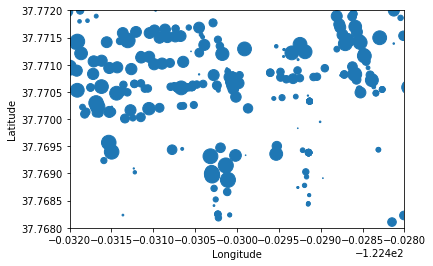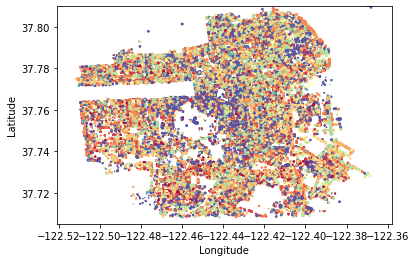Scatter plots are a beautiful way to display your data. Luckily, Pandas Scatter Plot can be called right on your DataFrame.

Scatter plots traditionally show your data up to 4 dimensions – X-axis, Y-axis, Size, and Color. Of course you can do more (transparency, movement, textures, etc.) but be careful you aren’t overloading your chart.

Pandas` DataFrame.plot.scatter()` will take your DataFrame and output a scatter plot. The default values will get you started, but there are a ton of customization abilities available.

```1. pd.DataFrame.plot.scatter(x=df['your_x_axis'],
y=df['your_y_axis'],
s=df['your_size_values'],
c=df['your_color_values'])```

This function is heavily used when displaying large amounts of data.

## Pandas Scatter Plot

The first question you always want to keep in mind when displaying data – What is the message I’m trying to say?

With this in mind, do not overload your charts. Make sure they are saying exactly what you want and nothing more. No fancy colors if you don’t need them, no exaggerated sizes that don’t provide value.

### Scatter Parameters

Before we get into the scatter plot specific parameters, keep in mind that Pandas charts inherit other parameters from the general Pandas Plot function. These other parameters will deal with general chart formatting vs scatter specific attributes. We recommend viewing these for full chart flexibility. We’ll use some in our example below.

• x: This where you specify a column name to be your X (horizontal) axis
• y: This where you specify a column name to be your Y (vertical) axis
• s: Size – How big do you want your points to be? You can specify
• Single number (scalar): This will set all of your points to the same size
• Column name: This will set your sizes per data point according to a value in a column.
• Array: This will set your data points size alternating between the values in your array. Ex: Passing [3,5] will set every other datapoint 3, then 5.
• c: Color – You can pass
• Single color – Either a hex string ‘#b31d59’ or ‘red’
• Array of colors – Setting your data points alternating between array values. Ex: [‘green’, ‘red’, ‘blue’] means pandas will color your points green, red, blue alternating.
• **kwargs: There are a huge number of extra parameters you could pass scatter. Check out the general parameters that come with all pandas charts here.

Here’s a Jupyter notebook with a few examples

In :
```import pandas as pd
```

### Pandas Scatter Plot¶

Not only can Pandas handle your data, it can also help with visualizations. Let's run through some examples of scatter plots. We will be using the San Francisco Tree Dataset. To download the data, click "Export" in the top right, and download the plain CSV.

Examples:

1. Default Scatter plot
2. Scatter Plot with specific size
3. Scatter plot with specific size and color
4. Extra customized scatter plot using the general DataFrame.plot() parameters

First, let's import our data

In :
```df = pd.read_csv("../data/Street_Tree_List.csv", parse_dates=['PlantDate']) # Importing our data, reading plant date as dates

# Feature Engineering
df['PlantYears'] = (pd.to_datetime('today') - df['PlantDate']) / pd.Timedelta(days=365) # Years since planting
df['qSpecies'] = df['qSpecies'].apply(lambda x: x.split(" ")) # Extracting the parent species

df = df[['Latitude', 'Longitude', 'PlantYears', 'qSpecies']] # Taking a subset of columns
df.dropna(subset=['PlantYears', 'Latitude'], inplace=True) # Dropping NA values.
```
Out:
LatitudeLongitudePlantYearsqSpecies
266637.776997-122.42477838.669693Ficus
267037.743793-122.4170064.362844Magnolia
267737.778292-122.42486838.677912Ficus
268137.744335-122.4386623.635952Tristaniopsis
268437.749705-122.4326184.485452Eriobotrya

### 1. Default Scatter plot¶

Let's start off by creating a regular scatter plot. Due to this dataset, I'll need to specify the bounds of the Y Axis as well. I'm using the Latitude and Longitude of each tree in SF as it's scatter points. This will show where in SF these trees are located.

The chart doesn't really look like much does it? However we can start to see the outline of San Francisco. Note: I had to set ylim ("Y Limit") in order to remove some outliers.

In :
```df.plot.scatter(x='Longitude',
y='Latitude',
ylim=(37.69, 37.82));
```### 2. Scatter Plot with specific size¶

Next up is to change the size of our points on our scatter plot. I first want to make them all the same size, but smaller. I'll do this by passing a scaler (single value) into the "s=" parameter.

In :
```df.plot.scatter(x='Longitude',
y='Latitude',
ylim=(37.69, 37.82),
s=.05);
```It's cool to see some of the streets start to come out with the smaller points!

Now let's say I wanted to make the points bigger or smaller, relative to each trees age. In order to do this, I'll start with a bit of feature engineering to extract the trees age in years.

Note: I'm also zooming in (by adjusting the x/y limits) to see the size differences better.

Check out the size differences now. The older treets are bigger.

Notice how I'm squaring the sizes, and dividing by 10. This is to help transform the values within 'PlantYears' into values that will make the data look good.

In :
```df.plot.scatter(x='Longitude',
y='Latitude',
ylim=(37.768, 37.772),
xlim=(-122.432, -122.428),
s=(df['PlantYears']**2)/10);
```### 3. Scatter plot with specific size and color¶

Now let's deal with some color. I want to color code each tree species in my dataset. In order to do this I need to generate a specific color for each tree depending on what species it is.

I wish pandas was a bit more forgiving when generating colors for labels, but oh well.

To do this I'm going to:

1. Import matpotlib & numpy and get a colormap (list of color values)
2. Create a Series (from a dictionary) corresponding each tree species with a random color (using a random state so you can copy)
3. Merging that series back onto the larger dataframe so I have a color value for each tree species.
In :
```# Step 1. Import Matpliblib and get a color map
import matplotlib
import numpy as np
np.random.seed(seed=30)

cmap = matplotlib.cm.get_cmap('Spectral') # Getting a list of color values.
```
In :
```# Step 2. Create a Pandas series (needed to merge) from a dictionary
# Passing a number betwen 0-1 into cmap will return a color to me
color_dict = pd.Series({k:cmap(np.random.rand()) for k in df['qSpecies'].unique()})

# Naming my series so I can merge it below. Only named series can be merged
color_dict.name = 'color_dict'
```
In :
```# Step 3. Merge your two datasets
df = pd.merge(df, color_dict, how='left', left_on='qSpecies', right_index=True)
```
In :
```df.plot.scatter(x='Longitude',
y='Latitude',
ylim=(37.705, 37.81),
s=(df['PlantYears'])/10,
c=df['color_dict']);
```Sweet! Check out how each tree species in our dataset is now a different color. It's cool to see how different neighborhoods have different densities of tree species.

### 4. Extra customized scatter plot using the general DataFrame.plot() parameters¶

Now lets go crazy and make our chart exactly how we want it. To do this I'll use a lot of other parameters from the general Pandas Plot function

In :
```df.plot.scatter(x='Longitude',
y='Latitude',
ylim=(37.705, 37.81),
s=(df['PlantYears'])/10,
c=df['color_dict'],
figsize=(9,8), # Setting the size of the plot
title="Trees In San Francisco", # Setting the title
xlabel='Longitude', # Labeling X Axis
ylabel='Latitude'); # Labeling Y Axis
```